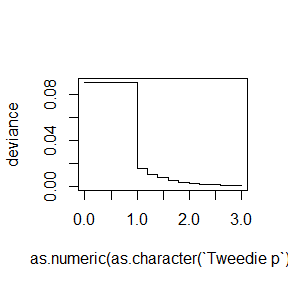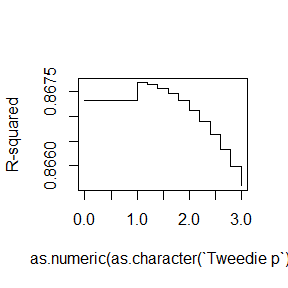# MetricsWeighted

#### 2020-01-07

library(MetricsWeighted)

## Introduction

The R package MetricsWeighted provides weighted versions of different machine learning metrics, scoring functions and performance measures as well as tools to use it within a dplyr chain.

## Installation

From CRAN:

install.packages("MetricsWeighted")

library(devtools)
install_github("mayer79/MetricsWeighted")

## Illustration

### Metrics, Scoring Functions and Performance Measures

Currently, the following metrics, scoring functions and performance measures are available:

• accuracy, recall, precision, f1_score, and classification_error: Typical binary performance measures derived from the confusion matrix, see e.g. (https://en.wikipedia.org/wiki/Precision_and_recall). Require binary predictions. Except for classification error, high values are better.

• AUC and gini_coefficient: Area under the receiver operating curve (ROC) and the closely related Gini coefficient. Actual values must be 0 or 1, while predictions can take any value (only their order is relevant). The higher, the better.

• deviance_bernoulli and logLoss: Further metrics relevant for binary targets, namely the average unit deviance of the binary logistic regression model (0-1 response) and logLoss (half that deviance). As with all deviance measures, smaller values are better.

• mse, mae, mape, rmse, and medae: Typical regression metrics (mean-squared error, mean absolute error, mean absolute percentage error, root-mean-squared error, and median absolute error). The lower, the better.

• deviance_tweedie: (Unscaled) average unit Tweedie deviance with parameter tweedie_p, see (Jorgensen 1997) and (https://en.wikipedia.org/wiki/Tweedie_distribution) for a reference of the underlying formula.

• deviance_normal, deviance_gamma, and deviance_poisson: Special cases of Tweedie. deviance_normal equals mean-squared error.

• elementary_score_quantile and elementary_score_expectile: Consistent scoring functions for quantiles and expectiles, see (Ehm et al. 2016).

They all take at least four arguments:

1. actual: Actual observed values.

2. predicted: Predicted values.

3. w: Optional vector with case weights.

4. ...: Further arguments.

#### Examples: Regression

# The data
y_num <- iris[["Sepal.Length"]]
fit_num <- lm(Sepal.Length ~ ., data = iris)
pred_num <- fit_num$fitted weights <- seq_len(nrow(iris)) # Performance metrics mae(y_num, pred_num) # unweighted #>  0.2428628 mae(y_num, pred_num, w = rep(1, length(y_num))) # same #>  0.2428628 mae(y_num, pred_num, w = weights) # different #>  0.2561237 rmse(y_num, pred_num) #>  0.300627 medae(y_num, pred_num, w = weights) # median absolute error #>  0.2381186 # Normal deviance equals Tweedie deviance with parameter 0 deviance_normal(y_num, pred_num) #>  0.09037657 deviance_tweedie(y_num, pred_num, tweedie_p = 0) #>  0.09037657 deviance_tweedie(y_num, pred_num, tweedie_p = -0.001) #>  0.09053778 # Poisson deviance equals Tweedie deviance with parameter 1 deviance_poisson(y_num, pred_num) #>  0.01531595 deviance_tweedie(y_num, pred_num, tweedie_p = 1) #>  0.01531595 deviance_tweedie(y_num, pred_num, tweedie_p = 1.01) #>  0.01504756 # Gamma deviance equals Tweedie deviance with parameter 2 deviance_gamma(y_num, pred_num) #>  0.002633186 deviance_tweedie(y_num, pred_num, tweedie_p = 2) #>  0.002633186 deviance_tweedie(y_num, pred_num, tweedie_p = 1.99) #>  0.002679764 deviance_tweedie(y_num, pred_num, tweedie_p = 2.01) #>  0.00258742 #### Examples: Binary classification # The data y_cat <- iris[["Species"]] == "setosa" fit_cat <- glm(y_cat ~ Sepal.Length, data = iris, family = binomial()) pred_cat <- predict(fit_cat, type = "response") # Performance metrics AUC(y_cat, pred_cat) # unweighted #>  0.9586 AUC(y_cat, pred_cat, w = weights) # weighted #>  0.9629734 logLoss(y_cat, pred_cat) # Logloss #>  0.2394547 deviance_bernoulli(y_cat, pred_cat) # LogLoss * 2 #>  0.4789093 ### Generalized R-squared Furthermore, we provide a generalization of R-squared, defined as the proportion of deviance explained, i.e. one minus the ratio of residual deviance and intercept-only deviance, see e.g. (Cohen 2003). By default, it calculates the ordinary R-squared, i.e. proportion of normal deviance (mean-squared error) explained. However, you can specify any different deviance function, e.g. deviance_tweedie with paramter 1.5 or the deviance of the binary logistic regression (deviance_bernoulli). #### Examples summary(fit_num)$r.squared
#>  0.8673123

# same
r_squared(y_num, pred_num)
#>  0.8673123
r_squared(y_num, pred_num, deviance_function = deviance_tweedie, tweedie_p = 0)
#>  0.8673123
r_squared(y_num, pred_num, deviance_function = deviance_tweedie, tweedie_p = 1.5)
#>  0.8675195

# weighted
r_squared(y_num, pred_num, w = weights)
#>  0.8300011
r_squared(y_num, pred_num, w = weights, deviance_function = deviance_gamma)
#>  0.8300644
r_squared(y_num, pred_num, w = weights, deviance_function = deviance_tweedie, tweedie_p = 2)
#>  0.8300644
r_squared(y_num, pred_num, deviance_function = deviance_tweedie, tweedie_p = 1.5)
#>  0.8675195

# respect to own deviance formula
myTweedie <- function(actual, predicted, w = NULL, ...) {
deviance_tweedie(actual, predicted, w, tweedie_p = 1.5, ...)
}
r_squared(y_num, pred_num, deviance_function = myTweedie)
#>  0.8675195

### Tidyverse

In order to facilitate the use of these metrics in a dplyr chain, you can try out the function performance: Starting from a data set with actual and predicted values (and optional case weights), it calculates one or more metrics. The resulting values are returned as a data.frame. Stratified performance calculations can e.g. be done by using do from dplyr.

#### Examples

require(dplyr)

# Regression with Sepal.Length as response
iris %>%
mutate(pred = predict(fit_num, data = .)) %>%
performance("Sepal.Length", "pred")
#>   metric    value
#> 1   rmse 0.300627

# Same
iris %>%
mutate(pred = predict(fit_num, data = .)) %>%
performance("Sepal.Length", "pred", metrics = rmse)
#>   metric    value
#> 1   rmse 0.300627

# Grouped by Species
iris %>%
mutate(pred = predict(fit_num, data = .)) %>%
group_by(Species) %>%
do(performance(., actual = "Sepal.Length", predicted = "pred"))
#> # A tibble: 3 x 3
#> # Groups:   Species 
#>   Species    metric value
#>   <fct>      <fct>  <dbl>
#> 1 setosa     rmse   0.254
#> 2 versicolor rmse   0.329
#> 3 virginica  rmse   0.313

# Customized output
iris %>%
mutate(pred = predict(fit_num, data = .)) %>%
performance("Sepal.Length", "pred", value = "performance",
metrics = list(root-mean-squared error = rmse))
#>                    metric performance
#> 1 root-mean-squared error    0.300627

# Multiple measures
iris %>%
mutate(pred = predict(fit_num, data = .)) %>%
performance("Sepal.Length", "pred",
metrics = list(rmse = rmse, mae = mae, R-squared = r_squared))
#>      metric     value
#> 1      rmse 0.3006270
#> 2       mae 0.2428628
#> 3 R-squared 0.8673123

# Grouped by Species
iris %>%
mutate(pred = predict(fit_num, data = .)) %>%
group_by(Species) %>%
do(performance(., "Sepal.Length", "pred",
metrics = list(rmse = rmse, mae = mae, R-squared = r_squared)))
#> # A tibble: 9 x 3
#> # Groups:   Species 
#>   Species    metric    value
#>   <fct>      <fct>     <dbl>
#> 1 setosa     rmse      0.254
#> 2 setosa     mae       0.201
#> 3 setosa     R-squared 0.469
#> 4 versicolor rmse      0.329
#> 5 versicolor mae       0.276
#> 6 versicolor R-squared 0.585
#> 7 virginica  rmse      0.313
#> 8 virginica  mae       0.252
#> 9 virginica  R-squared 0.752

# Passing extra argument (Tweedie p)
iris %>%
mutate(pred = predict(fit_num, data = .)) %>%
performance("Sepal.Length", "pred",
metrics = list(normal deviance = deviance_normal,
Tweedie with p = 0 = deviance_tweedie),
tweedie_p = 0)
#>               metric      value
#> 1    normal deviance 0.09037657
#> 2 Tweedie with p = 0 0.09037657

### Parametrized scoring functions

Some scoring functions depend on a further parameter $$p$$, e.g.

• tweedie_deviance and r_squared for deviance_function = deviance_tweedie: depends on tweedie_p,
• elementary_score_expectile, elementary_score_quantile: depend on theta.

It might be of key relevance to evaluate such function for varying $$p$$. That is where the function multi_metric shines.

#### Examples

ir <- iris
ir\$pred <- predict(fit_num, data = ir)

# Create multiple Tweedie deviance functions
multi_Tweedie <- multi_metric(deviance_tweedie, tweedie_p = c(0, seq(1, 3, by = 0.2)))
perf <- performance(ir, actual = "Sepal.Length", predicted = "pred",
metrics = multi_Tweedie, key = "Tweedie p", value = "deviance")
#>   Tweedie p    deviance
#> 1         0 0.090376567
#> 2         1 0.015315945
#> 3       1.2 0.010757362
#> 4       1.4 0.007559956
#> 5       1.6 0.005316008
#> 6       1.8 0.003740296
plot(deviance ~ as.numeric(as.character(Tweedie p)), data = perf, type = "s")# Same for Tweedie-R-squared
multi_Tweedie_r2 <- multi_metric(r_squared, deviance_function = deviance_tweedie,
tweedie_p = c(0, seq(1, 3, by = 0.2)))
perf <- performance(ir, actual = "Sepal.Length", predicted = "pred",
metrics = multi_Tweedie_r2, key = "Tweedie p", value = "R-squared")
plot(R-squared ~ as.numeric(as.character(Tweedie p)), data = perf, type = "s")